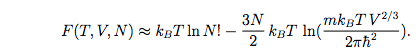# Canonical partition function of an ideal gas (unit analysis)

• Somali_Physicist

## Homework Statement

Basically the units of the Canonical Partition Function within the logarithms should be zero

## Homework Equations## The Attempt at a Solution

N here is a number so we ignore the left logarithms, applying a "Unit function " for the terms within the logarithm.

(Fu((mkbTV2/3)/(2πħ2)) ) -3/2 :
The above function can work on each individual portion and spit out the unit values , assuming all the operations act in the same way.

kbT => J (Thermal Energy)
m => Kg
ħ2 => J2s2
V2/3 => J2/3

Now ignoring the power to -3/2 =>
Kg*J*J2/3*s-2*J-2
=> kg*s-2*J-1/3
=> kg2/3s-8/3m-2/3
Now Even if we introduce -3/2 we don't acquire a unitless solution

#### Attachments

Your units for the volume V are wrong.

A useful combination to know is ##\hbar c = 197~{\rm eV\,nm}##. If you multiply the top and bottom by ##c^2## where ##c## is the speed of light, you get
$$\frac{(mc^2)(k_\text{B} T) V^{2/3}}{2\pi(\hbar c)^2}.$$ In that form, it's pretty easy to see that both the numerator and denominator have units of (energy x length)^2.

•Somali_Physicist
Your units for the volume V are wrong.

A useful combination to know is ##\hbar c = 197~{\rm eV\,nm}##. If you multiply the top and bottom by ##c^2## where ##c## is the speed of light, you get
$$\frac{(mc^2)(k_\text{B} T) V^{2/3}}{2\pi(\hbar c)^2}.$$ In that form, it's pretty easy to see that both the numerator and denominator have units of (energy x length)^2.
Volume ! i thought it was potential energy!
Gracias!

well for completeness
Kg * J * m3*2*3-1 *J-2*S-2
=> Kg*J-1*m2*s-2
=> J*J-1 => 1
Note - Kg*m2*s-2 = J